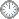# “执行定理”的证明(b)

This is an in-mail from TYUST.
新入の者--> What is going on ? (redirected)new

(接前: 28 25 24) “执行定理” (Th1.6) 的证明(b).
.
(我)在解读证明的过程中将时常指向如下图示:
.
a b c d
|  |  |  |
Theorem 1.6
|  |
1 2
.

---- 1. lct(X, B, |M|) ≥ lct(X, B, |A|).
---- 2. 上式右端 有正下界.
.

.

We want to apply Proposition 5.9, so we need to replace X with a Q-factorial one.
---- 整个定理的证明用到两个“主力”命题: 5.9 和 5.7.
---- 第二~四段及第五段开头只用到 5.9.
(第五段末尾才用到 5.7).
---- 5.9 假定 X 是 Q-factorial.

.
Since A is very ample and A ≤ r, X belongs to a bounded family of varieties depending only on d, r.
---- 此处体现出条件 b 是有界族的一个充分条件！
(这或许提示了条件 b 的来由).
---- 但不知, 此处是否用到 (X, B) eps-lc 的假定(?).
.
a  b
?|   |
para2.2
|
3(有界族)
.

.
Thus we can pick a resolution φW --> X so that if Γ is the sum of the exceptional divisors, then (W, Γ) belongs to a bounded family of pairs depending only on d, r.
---- 取映射φ: W --> X...
(取 resolution 是常规手法, 但何时该这样做?)
---- 令 Γ = ΣEi, 其中Ei 是 exceptional divisor.
(ΣEi 记作 Eφ 更方便).
---- 则 (W, Γ) 属于有界配对族.
.
3
|
para2.3
|   |
4  5
.

---- 4. resolution φ: W --> X.
---- 5.  (W, Γ) ∈ 有界(配对)族.
(若 X 属于有界族, 则 像配对 属于有界配对族)
.

(比如, “本征像配对”, 它由Γ的特定构造刻画).
.
Let X' be a minimal model of (W, (1 - eps/2)Γ) over X.
---- 此处体现出 本征像配对 的可配置性
---- 即可以给 Γ 配置系数 (按某种需要).
---- minimal model 须有 MMP, 此处未提及.(?)
.
5'
|
para2.4
|
6
.

---- 5' 即 (W, (1 - eps/2)Γ), 可看做 5 的 argumentation 版.
---- 6  即 X', 该是 W 的像(或 X 的二重像).
.

.
Since A, A - B, and Kx + B are R-Cartier, Kx is Q-Cartier, hence (X, 0) is eps-lc.
---- 三个量 R-Cartier 未提及来由.
(这是 “Since 体”, 来由得自行推导?).
---- Kx 系Q-Cartier是前半句推出的, 还是追加的?
(姑且当作是追加的).
---- 得到结果 (X, 0) eps-lc 的用意似在下一句.
.
e
|
para2.5
|
7
.

---- e 即此句的条件部分(含4个“分量”)
(e 的来由待考?)
---- 7 即 (X, 0) eps-lc.
.

---- 特意考虑 (X, 0), 应该是为了规避掉 B.
.
We can write Kw + (1 - eps/2)Γ =φ*Kx + E where E is effective with the same support as Γ.
---- 此公式为“锻法”, 或将造相.
---- 右端的 φ*Kx  体现出规避 B 的效用.
---- E 的描述可能是“锻公式”本身自带的.(?)
.
5'  7
|   |
para2.6
|
8
.

---- 8 即锻公式(含E的描述).
.

.
By the negativity lemma, E is contracted over X', hence X' --> X is just a Q-factorialisation of X.
---- 由负性引理, E 在 X' 之上受压缩, 从而 X' --> X 只是 X 的 Q-因式化.
.
f  8
|   |
para2.7
|
9
|
10
.

---- f: 负性引理(待查?)
(显然跟锻公式有关; Γ的系数缘于此).
---- 9: E 在 X' 之上受压缩.
---- 10X' --> X 是 X 的 Q-因式化.
.

.

符号大全上下标.|| 常用：↑↓ πΓΔΛΘΩμφΣ∈  ∪ ∩ ⊆ ⊇ ⊂ ⊃ ≤ ≥ ⌊ ⌋ ⌈ ⌉ ≠ ≡ ⁰ ¹ ² ³ ᵈ ₀ ₁ ₂ ₃ ᵢ .

#### Glossary(AG)

*

Introduction
....
....
...

http://blog.sciencenet.cn/blog-315774-1195897.html

## 全部精选博文导读

GMT+8, 2020-9-30 16:57